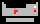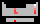▸▸
• 🇬🇧 Iodine
• 🇨🇳 碘
• 🇳🇱 Jood
• 🇫🇷 Iode
• 🇩🇪 Iod
• 🇮🇱 יוד
• 🇮🇹 Iodio
• 🇯🇵 ヨウ素
• 🇵🇹 Iodo
• 🇷🇺 Иод
• 🇪🇸 Yodo
• 🇸🇪 Jod

# Iodine: radii of atoms and ions53I

One measure of size is the element-element distance within the element. It is not always easy to make sensible comparisons between the elements however as some bonds are quite short because of multiple bonding (for instance the O=O distance in O2 is short because of the the double bond connecting the two atoms. The bond length in II is: 266.6pm.

There are several other ways ways to define radius for atoms and ions. Follow the appropriate hyperlinks for literature references and definitions of each type of radius. All values of radii are given in picometres (pm). Conversion factors are:

• 1 pm = 1 × 10‑12 metre (meter)
• 100 pm = 1 Ångstrom
• 1000 pm = 1 nanometre (nm, nanometer)

The size of neutral atoms depends upon the way in which the measurement is made and the environment. Follow the appropriate hyperlinks for definitions of each radius type. The term "atomic radius" is not particularly helpful although its use is widespread. The problem is its meaning, which is clearly very different in different sources and books. Two values are given here, one is based upon calculations and the other upon observation - follow the appropriate link for further details.

The following are calculated values of valence shell orbital radii, Rmax

### References

The Rmax values for neutral gaseous element valence orbitals are abstracted from reference 1.

1. J.B. Mann, Atomic Structure Calculations II. Hartree-Fock wave functions and radial expectation values: hydrogen to lawrencium, LA-3691, Los Alamos Scientific Laboratory, USA, 1968.

I(VII)4-coordinate, tetrahedral56I(V)6-coordinate, octahedral109I(VII)6-coordinate, octahedral67